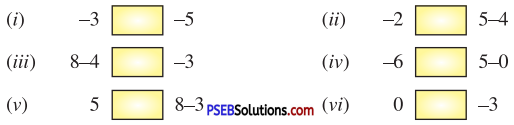# PSEB 7th Class Maths Solutions Chapter 1 Integers Ex 1.1

Punjab State Board PSEB 7th Class Maths Book Solutions Chapter 1 Integers Ex 1.1 Textbook Exercise Questions and Answers.

## PSEB Solutions for Class 7 Maths Chapter 1 Integers Ex 1.1

1. Use the appropriate symbol >, <, = to fill in the blanks(i) -3  -5
(ii) – 2  5-4
(iii) 8-4  – 3
(iv) – 6  5-0
(v) 5  8-3
(vi) 0  – 3.

2. Arrange the following integers in ascending order.

Question (i)
– 2, 12, – 43, 31, 7, – 35, – 10
Given positive integers are 12, 31, 7
Ascending order is 7 < 12 < 31
Given negative integers are – 2, – 43, – 35, -10
Ascending order is – 43 < – 35 < – 10 < -2.
Hence, all given integers in ascending order are :
– 43 < – 35 < -10 < – 2 < 7 < 12 < 31.
i.e. – 43, – 35, – 10, – 2, 7, 12, 31.

Question (ii)
– 20, 13, 4, 0, – 5, 5
Given positive integers are 13, 4, 5
Ascending order is 4 < 5 < 13
Given negative integers are – 20, – 5
Ascending order is -20 < – 5.
Hence all given integers in ascending order are :
– 20 < – 5 < 0 < 4 < 5 < 13
i.e. – 20, – 5, 0, 4, 5, 133. Arrange the following integers in descending order.

Question (i)
0, – 7, 19, – 23, – 3, 8, 46
Given positive integers are 19, 8, 46
Descending order is 46 > 19 > 8
Given negative integers are – 7, – 23, – 3
Descending order is – 3 > – 1 > – 23
Hence, all given integers in descending order are :
46 > 19 > 8 > 0 > – 3 > – 7 > – 23
i.e. 46, 19, 8, 0, – 3, – 7, – 23

Question (ii)
30, – 2, 0, – 6, – 20, 8.
Given positive integers are 30, 8
Descending order is 30 > 8
Given negative integers are – 2, – 6, – 20
Descending order is-2 > -6 > -20
Hence all given integers in descending order are :
30 > 8 > 0 > -2 > – 6 > – 20
i.e. 30, 8, 0, – 2, – 6, – 20

4. Evaluate :

Question (i)
30 – | -21 |
30 – | -21 | = 30 – 21
[∵ | – 21 | = 21]
= 9

Question (ii)
| -25 | – | -18 |
| -25 | – | – 18 | = 25 – 18
[∵ | -25 | = 25 and | – 18 | = 18]
= 7

Question (iii)
6 – | -4 |
6 – | -4 | = 6 – 4
[∵ | – 4 | = 4]
= 2

Question (iv)
| – 125 | + | 110 |
| – 125 | + | 110 | = 125 + 110
[∵ | -125 | = 125 and | 110 | = 110]
= 2355. Fill in the blanks :

Question (i)
0 is greater than every …………… integer.
Negative

Question (ii)
Modulus of a negative integer is always ……………
Positive

Question (iii)
The smallest positive integer is ……………